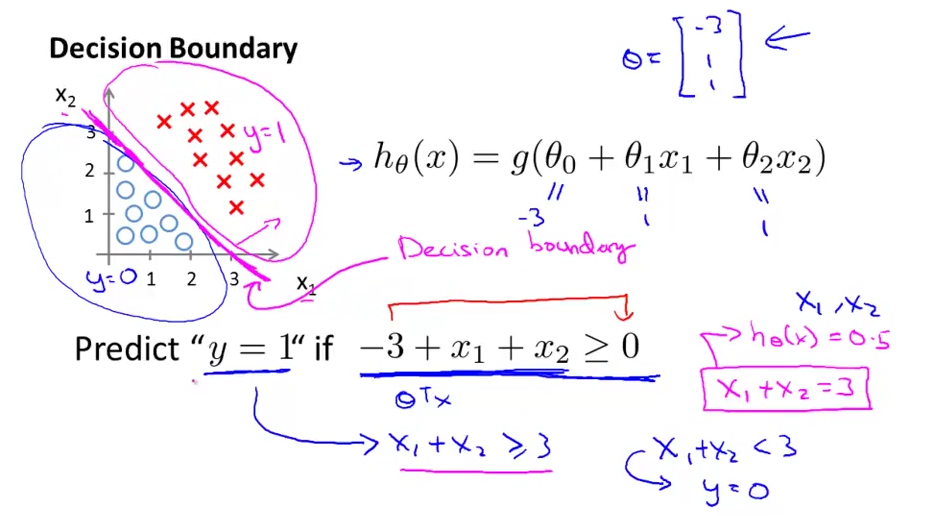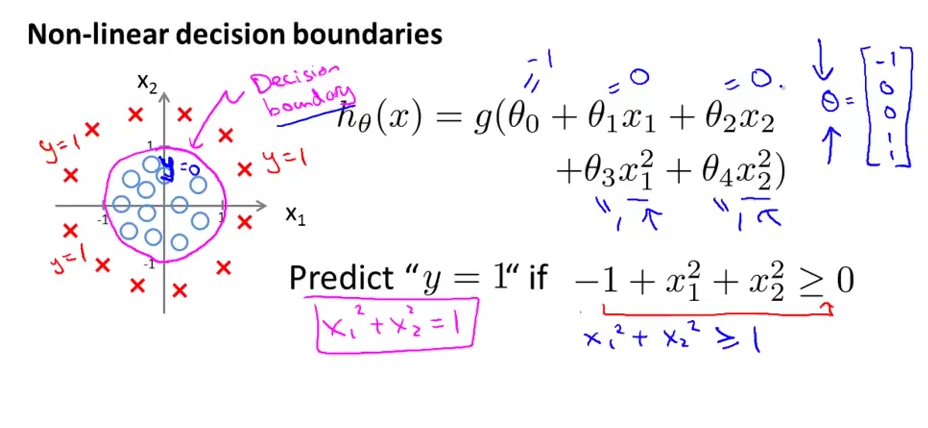Factorization Machine 主要目标是: 在数据稀疏的情况下解决组合特征的问题

## 推导

\begin{align}
\hat y = \omega_0 + \sum_{i=1}^{n}\omega_{i}x_{i}
\end{align}

\begin{align}
\hat y = \omega_0 + \sum_{i=1}^{n}\omega_{i}x_{i} + \sum_{i=1}^{n}\sum_{j=i+1}^{n}\omega_{ij}x_{i}x_{j}
\end{align}

\begin{align}
W = VV^{T}
\end{align}

It is well known that for any positive definite matrix W, there exists a matrix V such that W =V · Vt provided that k is sufficiently large. This shows that a FM can express any interaction matrix W if k is chosen large enough. Nevertheless in sparse settings, typically a small k should be chosen because there is not enough data to estimate complex interactions W. Restricting k – and thus the expressiveness of the FM – leads to better generalization and thus improved interaction matrices under sparsity

\begin{align}
\hat y & = \omega_0 + \sum_{i=1}^{n}\omega_{i}x_{i} + \sum_{i=1}^{n}\sum_{j=i+1}^{n} <v_{i}, v_{j}>x_{i}x_{j}
\end{align}

\begin{align}
\sum_{i=1}^{n}\sum_{j=i+1}^{n} <v_{i}, v_{j}>x_{i}x_{j} & = \frac{1}{2}\sum_{i=1}^{n}\sum_{j=1}^{n} <v_{i}, v_{j}>x_{i}x_{j} - \frac{1}{2}\sum_{i=1}^{n} <v_{i}, v_{i}>x_{i}x_{i} \\
& =\frac{1}{2} (\sum_{i=1}^{n}\sum_{j=1}^{n}\sum_{f=1}^{k}v_{i,f}v_{j,f}x_{i}x_{j} - \sum_{i=1}^{n}\sum_{f=1}^{k}v_{i, f}v_{i,f}x_{i}x_{i}) \\
& = \frac{1}{2}\sum_{f=1}^{k}((\sum_{i=1}^{n}v_{i,f}x_{i})(\sum_{j=1}^{n}v_{j,f}x_{j}) - \sum_{i=1}^{n} v_{i,f}^2 x_i^2)\\
& = \frac{1}{2}\sum_{f=1}^{k}((\sum_{i=1}^{n}v_{i,f}x_{i})^2 - \sum_{i=1}^{n} v_{i,f}^2 x_i^2)\\
\end{align}

\begin{align}
\hat y = \omega_0 + \sum_{i=1}^{n}\omega_{i}x_{i} + \frac{1}{2}\sum_{f=1}^{k}((\sum_{i=1}^{n}v_{i,f}x_{i})^2 - \sum_{i=1}^{n} v_{i,f}^2 x_i^2)
\end{align}

## 训练

### 回归问题

\begin{align}
loss^{R}(\hat y, y) = \frac{1}{2m}\sum_{i=1}^{m}(\hat y^{(i)} - y^{(i)})^2
\end{align}

### 分类问题

CTR预测本质上就是一个二元分类问题, 结果为点击的概率。分类问题中 logloss 做损失函数, $$\sigma$$ 为 sigmoid函数

\begin{align}
loss^{C}(\hat y, y) = \sum_{i=1}^{m} -ln\sigma(\hat y^{(i)}y^{(i)})
\end{align}

### 梯度下降

\begin{align}
\theta = \theta - \alpha\frac{\partial{loss}}{\partial\theta}
\end{align}

## 应用

• 适用于CTR预估这种稀疏矩阵的情况
• 做好特征工程后就不用考虑组合特征了, 如果是在LR模型中添加组合特征, 则需要极其深刻的领域知识
• 在线上进行预测时候速度快, 模型训练也快

• libFM
• SVDFeature
• difacto

]]>

## 决策边界

sigmoidz函数
$$h(z) = \frac {1} {1 + e^{-z}}$$$$z = \omega^{T} x + \omega_{0}$$

$$h(z) = p(y=1 |x;\omega;\omega_{0})$$

$$x_1$$ $$x_2$$, 分类数为2, 分别用 圆圈红叉 表示, 可画成下面的图## 损失函数

$$Loss = \frac {1} {2m} \sum_{i=1}^{m}(y_{i} - \frac {1} {1 + e^{-\omega^{T}x}})^2$$\begin{align}
P_{y=1} = \frac {1} {1 + e^{-w^Tx}}
\end{align}

\begin{align}
P(y_{i}|x_{i}) = p^{y_i}(1-p)^{1-y_i}
\end{align}

\begin{align}
P_{total} &= P(y_1|x_x) P(y_2|x_2) P(y_3|x_3) … P(y_n|x_n) \\
&= \prod_{i=1}^{n} p^{y_i} (1-p)^{1-y_i}
\end{align}

\begin{align}
F(\omega) &= ln(P_{total}) \\
&= ln(\prod_{i=1}^{n} p^{y_i} (1-p)^{1-y_i}) \\
&= \sum_{i=1}^{n}ln(p^{y_i}(1-p)^{1-y_i}) \\
&= \sum_{i=1}^{n}(y_iln(p) + (1-y_i)ln(1-p))
\end{align}

$$\omega^{*} = \mathop{argmax}_{\omega} F(\omega)$$

## 梯度下降

\begin{align}
\nabla{F(\omega)} &= \nabla (\sum_{i=1}^{n}(y_iln(p) + (1-y_i)ln(1-p)))\\
&= \sum_{i=1}^{n}(y_i ln’(p) + (1-y_i)ln’(1-p)) \\
&= \sum_{i=1}^{n}(y_i\frac{1}{p}p’ + (1-y_i)\frac{1}{1-p}(1-p)’) \\
&= \sum_{i=1}^{n}(y_i\frac{1}{p}p(1-p)x_i - (1-y_i)\frac{1}{1-p}p(1-p)x_i) \\
&= \sum_{i=1}^{n}(y_i(1-p)x_i - (1-y_i)px_i)\\
&= \sum_{i=1}^{n}(y_i - p)x_i
\end{align}

\begin{align}
\omega_{t+1} = \omega_{t} + \eta N (y_i - \frac {1} {1 + e^{-\omega^Tx_i}})
\end{align}

\begin{align}
\omega_{t+1} = \omega_{t} + \eta (y_i - \frac {1} {1 + e^{-\omega^Tx_i}})
\end{align}

## 代码实现

https://github.com/Tara-X/algo/tree/master/LR

## 与SVM/感知机的区别

• 感知机模型将分离超平面对数据分割，寻找出所有错误的分类点，计算这些点到超平面的距离，使这一距离和最小化，也就是说感知机模型的最优化问题是使得错误分类点到超平面距离之和最小化。
• 逻辑斯蒂回归是将分离超平面作为sigmoid函数的自变量进行输入，获得了样本点被分为正例和反例的条件概率，然后用极大似然估计极大化这个后验概率分布，也就是说逻辑斯蒂回归模型的最优化问题是极大似然估计样本的后验概率分布。
• 支持向量机的最优化问题是最大化样本点到分离超平面的最小距离。

]]>

## 基本概念

• 代价函数/损失函数 (Cost fuction)

:线性回归的目标是让 假设函数与训练集尽量的拟合，使得代价函数最小,前面的 $$1/2$$的作用是因为平方误差函数求导之后正好抵消,使得在梯度下降里面的偏导数项系数正好为1

$$J(\theta_0, \theta_1) = \frac{1}{2m} \sum_{i=1}^{m}(h_\theta(x^{(i)}) - y^{(i)})^2$$

## 梯度下降算法

### 梯度下降公式$$\theta_j := \theta_j - \alpha\frac{\partial}{\partial\theta_j}J(\theta_0,\theta_1)$$

### 理解梯度

• 梯度即变化率最大的那个方向导数
• 偏导数是一类特殊的方向导数
• 求得某点的各方向偏导数, 可以构成一个向量, 沿着该向量变化最大, 即方向导数

## 多元线性回归

\begin{align}
\hat{y} = \omega_{0} + \sum_{i=1}^{k}\omega_{i}x_{i}
\end{align}

\begin{align}
loss^{R} = \frac{1}{2m}\sum_{i=1}^{m}(\hat{y}^{(i)} - y^{(i)})^2
\end{align}

\begin{align}
\frac{\partial{loss^{R}}}{\partial\theta} = \begin{cases}
\frac{1}{m}\sum_{i=1}^{m}(\hat{y}^{(i)} - y^{(i)}) & \text{if } \theta \text{ equals to } \omega_{0} \\
\\
\\
\frac{1}{m}x_{t}\sum_{i=1}^{m}(\hat{y}^{(i)} - y^{(i)}) & \text{if } \theta \text{ equals to } \omega_{t}
\end{cases}
\end{align}

• Batch Gradient Descent (BGD) 批量梯度下降, 每次迭代使用全部训练数据
• Stochastic Gradient Descent (SGD) 随机梯度下降, 每次仅仅选取一个样本来求梯度
• Mini-Batch Gradient Descent (MBGD) 小批量梯度下降，每次抽取x个样本进行训练

## 代码实现

https://github.com/Tara-X/algo/tree/master/linear

]]>

## 算法描述

### 算法特性

• 平衡性: 尽可能的将记录hash到所有节点当中, 最大化利用空间
• 单调性: 哈希的结果应能够保证原有已分配的内容可以被映射到原有的或者新的缓冲中去，而不会被映射到旧的缓冲集合中的其他缓冲区, 可以这么理解, 在增加一个节点之后, 原有的hash结果要么不迁移, 要么迁移到新的节点, 不会迁移到旧的节点中, 所以取模的那个方法, 增加节点之后很大一部分的key都会重新映射到原来的缓存系统的其他节点当中, 故不符合单调性, 在P2P系统中常用的DHT也用到了一致性Hash算法, 缓冲的变化等价于Peer加入或退出系统，这一情况在P2P系统中会频繁发生，因此会带来极大计算和传输负荷。单调性就是要求哈希算法能够应对这种情况。
• 分散性: 分布式环境中用户可能看不到所有的节点, 所以可能导致相同记录映射到不同节点上, 这种情况显然不太好, 分散性就是定义上述情况发生的严重程度, 应该尽量降低分散性
• 负载: 既然不同的终端可能将相同的内容映射到不同的分片节点中，那么对于一个特定的节点而言，也可能被不同的用户映射为不同的内容, 好的hash算法应该能够尽量降低节点负载
• 平滑性: 缓存服务器数量能够和记录数量的改变能够保持一致

### 算法实现

1. 根据(ip,port,mac等)求出节点的hash值, 分布在0-2^32的圆环上2. 如果有一个写入缓存的请求，其中Key值为K，计算器hash值Hash(K)， Hash(K) 对应于图 – 1环中的某一个点，如果该点对应没有映射到具体的某一个机器节点，那么顺时针查找，直到第一次找到有映射机器的节点，该节点就是确定的目标节点，如果超过了2^32仍然找不到节点，则命中第一个机器节点。比如 Hash(K) 的值介于A~B之间，那么命中的机器节点应该是B节点
3. 如果增加一个节点, 会初始化该节点到现有的环上, 比如加入了节点F, 初始该节点后集群状态如下那么只有C-F之间的区域的数据会出现节点不命中的情况, 将该区域的数据rebalance即可
4. 如果将F节点去掉, 那么还是只有C-F之间的区域数据会收到影响, 按照算法只要将F节点数据挪到D节点上即可

## 工程应用

### ShardedJedis

Jedis中使用ShardedJedis实现了集群特性(redis3的redis cluster也原生支持了), 实现一致性hash的主要思路是:

1. 虚拟节点采取TreeMap存储, 这样就能通过tailMap方法来实现环的特性

## 参考

]]>

2017年中总结 http://stackbox.cn/2017-07-2017-mid-schedule/ 2017-07-25T09:57:04.000Z 2018-12-17T11:05:09.253Z

• 一开始的时候做的是一个 主播榜单类似的东西, 首先这个花费了大量的人力物力, 但其实并没有产生很好的效果, 我想总结起来原因有以下几点
• 榜单这个东西只是数据的极其粗糙的处理, 并没有发掘出更深入的价值
• 再着, 基于某项新事物赚钱的前提应该是大趋势上依赖于这个新事物的利益相关方都赚到钱了, 比如微信公众号, 对于直播来讲, 各个平台亏得要死, 除了公会主播拿了大部分钱, 真没看到什么第三方赚到大钱, 在整体形势上都看不到未来的话, 基于直播做To B类产品盈利只是空中楼阁
• 每当一个新的媒介形式出现之后, 目前来看差不多都是得靠着广告主爸爸来养着, 对于直播这种新兴的媒介, 它只是在占据人们的时间上来说很有优势, 但是传播性上不及微信微博, 精细程度上平均水平又很难和优酷爱奇艺相比, 广告预算只有可能是一些比较激进的并且财大气粗的广告主往直播上扔一点点, 没有很大体量的广告预算, 可以说营销这套体系根本就玩不转
• 然后又做了一个公会运营管理工具, 这个东西还算对公会有用, 但是这个项目权当是公益了, 因为这个系统加了一个直播弹幕的爬虫及分析系统, 还是略有一点复杂的
• 然后算是自暴自弃的搞了一个平台demo出来, 算是结束了这个方向的尝试

• 出去旅游两次
• 抽时间考完驾照
• 学英语
• 阅读一些比较重的代码, 比如spring, 有大型项目的建模能力
• numpy/pandas能够熟练使用
• 拜厄跟车尔尼599进度都达到一半
• 博客两周更新一篇（数据&项目设计方向）
• 口头表达能力, 尽量自己在家没事练习讲Slide
• lintcode刷一遍
• 至少看五本书
• 看一下外面的机会
]]>
2017年中总结以及下半年规划

### Supervisor

environment=AIRFLOW_HOME=xxxxxxxxxx

### AMPQ库

celery提供了两种库来实现amqp, 一种是默认的kombu, 另外一个是librabbitmq, 后者是对其c模块的绑定, 在1.8.1版本中, 使用的kombu的时候会出现scheduler自动断掉的问题, 这个应该是其对应版本4.0.2的问题, 当切成librabbitmq的时候, server 与 scheduler运行正常, 但是worker的从来不consume任务, 最后查出原因: Celery4.0.2的协议发生了变化但是librabbitmq还没有对应修改, 解决方法是, 修改源码里的 executors/celery_executor.py文件然后加入参数

### RabbitMQ连接卡死

BROKER_POOL_LIMIT=0 //不使用连接池

### RabbitMQ中的queue数量过多问题

celery为了让scheduler知道每个task的结果并且知道结果的时间为 O(1) , 那么唯一的解决方式就是给每一个任务创建一个UUID的queue, 默认这个queue的过期时间是1天, 可以通过更改celery_executor.py的参数来调节这个过期时间

]]>
Airflow是一个基于DAG(有向无环图)的任务管理系统

• ver: 版本信息
• serial: supervisor给事件的编号, 第一个事件为1, 之后事件编号递增
• eventpool: 产生event的event_listener名字
• poolserial: 与serial不同的是, 由于可以有多个eventpool,而且eventpool可以检测的范围事件范围可以不同， 这个poolserial是相对某个eventpool的编号
• eventname: supervisor 标准定义的事件状态

• processname: 触发事件的applicaiton名称
• groupname: 触发事件的application的组名称
• from_state: 事件触发状态之前的那个状态
• pid: 进程id

## 注意事项

1. 在配置文件中配置event_listener的时候, 需要配置一个 events 的选项, 用以表明只监听某些类型的事件, 可以设置多个事件类型
2. 监听器的处理事件流程为: readline()读取head -> 读取固定长度的data -> 输出状态信息 所以尽量避免在其中使用 print 等标准输出,,否则会破坏协议的完整性导致监听器失效, 如果想查看输出日志可以用文件或者网络传输等方式
3. 测试代码
]]>

## CasperJS

CasperJS是一个基于PhantomJS的工具套件, 相比原生的PhantomJS使用起来更人性化, 比如可以通过下面的代码来对网页截图

## 字体问题

]]>

Gephi是一款开源免费跨平台基于JVM的复杂网络分析软件, 其主要用于各种网络和复杂系统, 特别是在处理网络关系数据这方面很有优势,下面是两个不错的例子## Update:

1. 关于gexf文件的生成, 可以用这个python库: https://github.com/paulgirard/pygexf
2. 纯前端的话, sigma.js 提供了插件 https://github.com/jacomyal/sigma.js/tree/master/plugins/sigma.layout.forceAtlas2 来实现力导向算法

THE END

]]>
Gephi是一款开源免费跨平台基于JVM的复杂网络分析软件, 擅长处理图数据
PathFilter无法生效 http://stackbox.cn/2015-07-hdfs-pathfilter-not-work/ 2015-07-15T02:06:42.000Z 2018-12-17T11:06:49.249Z 最近再写一个MapReduce的时候出现了一个诡异的问题, PathFilter无法生效, 具体描述如下:

1. 代码参考的是另外一个项目(用的是SequenceFileInputFormat),其PathFilter能正常工作
2. 我写的这个MR用的公司的一个CombineFileInputFormat, 虽然设置了Filter但是程序运行的时候PathFilter甚至都没实例化。

1. CombineFileInputFormat: 重写了getSplits方法, 但是在重写的方法里调用了 super.listStatus(job), 所以PathFilter正常
2. SequenceFileInputFormat: 重写了listStatus方法, 但是在重写方法里调用了 super.listStatus(job), 所以PathFilter也依然正常
3. 那么再看看自己写的那个InputFormat, 也重写了getSplits方法, 但是获取block status是用 getInputPaths获取路径然后手动获取status的, 根本就没管 父类的listSt方法，因此导致PathFilter失效

THE END

]]>
HDFS中PathFilter无法生效的问题

Update @ 2016.03.02: 此文描述的工程构建方式已经有些复杂了(原生SpringMVC), 目前比较流行的方式是使用SpringBoot(附带oauth2等项目)构建

## 解决思路

• 对于平台类API来说,其目标用户一般是开发者, 诸如饿了么OpenApi或者 Pusher.com 这类服务,每次调用都是独立的, 无需保存状态信息, 数据权限和功能权限可以通过 AppId 这类唯一标识符来进行区分。安全上通过 auth_signature 的方式来进行校验。具体算法可以参见上面提到的两个文档。

• 如果目标对象是那些APP, 怎么办呢? , 刚工作那会解决这种需求的方法十分暴力:把用户名密码保存在app本地,调用接口的时候把用户名密码传过去做校验, 没有优雅性可言。目前来讲,在写Mobile API时, 直接使用 Oauth2 来处理权限问题是一种比较常用的方法。Oauth2 看起来略复杂,但其最终目的是获取一个 访问令牌 , 获取令牌的模式一共有四种.

1. 授权码: 例子有微博第三方登陆,流程为: 第三方网站 -> 跳转到微博让用户选择是否授权 -> 用户授权并通过回调返回第三方一个授权码 -> 第三方根据授权码向微博申请访问令牌 -> 微博返回访问令牌
2. 隐式授权: 流程为: 跳转到授权页面 -> 授权成功之后回调返回访问令牌
3. 密码模式: 流程为: 发送一个带用户名密码参数的请求(并附带Http Basic Authorization) -> 返回一个访问令牌
4. 客户端模式: 这个方式很有意思,在这种模式下, 是以客户端的名义而不是以用户的名义进行令牌申请, 权限上并没有区分,也就不存在授权问题了, 流程为: 向认证服务器发起请求 -> 以某种方式验证客户端的方式(比如根据appId,appSecret) -> 返回访问令牌

## 工程实践

• 配置spring-security

• 配置oauth2

The grant types supported by the AuthorizationEndpoint can be configured via the AuthorizationServerEndpointsConfigurer. By default all grant types are supported except password (see below for details of how to switch it on). The following properties affect grant types:

## 其他策略

• JWT(Json Web Tokens) 目前还是一份草案, 与Oauth2项目在服务器端配置上更简单些,目前在一些使用 Angular, Ember的单页面应用中已经被使用。JWT在passport和spring-security中都能够支持。

• CAS for Mobile, CAS是一个在写web项目时常用的单点登录服务器, 它也能够支持Rest API ,不过在客户端的处理比较麻烦,不过已经有了第三方的repo能够支持移动端CAS Android / iOS

]]>

# 分析

An attempt is made to read as many as len bytes, but a smaller number may be read.

1. 硬件上的buffersize比较小
2. 网络的IO比较慢
3. 文件是分布式的,组合在一起时需要花费一些时间

THE END

]]>
IO好复杂系列
maven大坑 http://stackbox.cn/2015-05-dammit-maven/ 2015-05-10T16:53:31.000Z 2018-12-17T11:06:32.552Z 乱码

• 登陆提交的表单会自动加一串奇奇怪怪的乱码
• Constant变量中的中文在当成message放在json中也会出现乱码

## mybatis代理失效

• 比较暴力的方法是把xml,properties 都放到src/main/resources
• 本着较少改动的原则,给pom.xml添加如下配置

## 其他

THE END

]]>
Damn it,maven！
Build Your Own Cas Service - Pro http://stackbox.cn/2015-01-build-your-own-cas-service-pro/ 2015-01-06T05:21:17.000Z 2018-12-17T11:09:10.064Z 示例代码: https://github.com/superalsrk/modify-jasig-cas ,以下所有描述都基于版本 3.5.2.1

## Generally Design

webapp module的pom为pom.xml

## Auth Module

### 自定义Credentials

Credentials是一个用户凭证, 可以理解为一个简易的pojo, 只要实现Credentials接口即可，我们的自定义凭证中除了用户名密码，还加了一个字段 product : String, 表明要登录的产品类型

1 . 在登录表单增加product字段,具体操作详见下个Section

### 自定义Handler

1 . 如果要在前台显示一个 权限不足 的信息, 只需在Handler里throw一个自定义的 AuthenticationException 即可
2 . support 接口用来声明handler是否支持某种类型的凭证
3 . 修改 /WEB-INF/deployConfigContext.xml ，进行handler的配置

### 自定义Resolver

Resolver是一个Credentials 到 Principal的转换器， 其中Principal其实是javaEE中就已经定义好的

1 . 修改 /WEB-INF/deployConfigContext.xml ，进行Resolver的配置

2 . resolver可以返回一个Principal, 个人觉得比较好用的方式是返回一个 #SimplePrincipal# ,除了用户的user信息外，还可以返回一个 AttrMap，不过需要参考下章进行Resolver视图的修改

##Web Module

### 自定义返回用户信息

1 . 在resolver中虽然返回了更多Attr，不过默认的Resolver视图不支持返回更多属性，需要对 protocol/2.0/casServiceValidationSuccess.jsp 页面进行扩展.

2 . 在client端，使用如下代码就可以获取多余属性

3 . 注意把deployerConfigContext.xml中 serviceRegistryDao全部删掉(cas),参考资料

### CAS退出功能

1. 如果只是退出应用，那么在此访问页面的时候，cas-client又会向cas-server端进行请求验证,然后自动登录,所以同时退出cas和应用即可
2. 修改 cas-servlet.xml , 在 logoutController 的bean中增加属性 p:followServiceRedirects=”true”
3. 假如应用已经有一个退出controller，此contoller用来清空session,那么链接 http://cas.example.org/logout?service=http://localhost:8080/logout 便可以正常退出

THE END

]]>

Build Your Own Cas Service - Basic http://stackbox.cn/2015-01-build-your-own-cas-service-basic/ 2015-01-06T05:21:16.000Z 2018-12-17T11:09:00.844Z 预备知识

JASIG有以下几个比较重要的接口

• Credentials 用户认证凭证, CAS的默认凭证只有用户名密码，所以如果想在认证的时候除了用户名密码外还要验证产品信息，就要自定义一个Credentials了，下面的Handler和Resolver都有一个support接口，用来判断是否支持处理某种类型的Credentials

• AuthenticationHandler 前台页面提交登录信息后，此接口判断登录信息是否能认证通过,接口会抛出一个AuthenticationException异常，用以在上层代码中catch并在前台页面显示错误信息

• AuthenticationException 在authentication阶段可能会抛出异常，抛出的异常信息可以前台页面中进行展示

## CAS部署与配置

### 无https配置

• 修改 /WEB-INF/deployerConfigContext.xml， 设置安全属性

THE END

]]>
JASIG-CAS基础配置,以及对几个核心接口的分析

## 原理

jQuery内置提供的jsonp功能,最简单的使用方式如下:

## 备注

JSONP是一个非标准的规范，其优点在于浏览器兼容性，而且由于发展

1. 使得rest风格的api不再那么优雅
2. 安全问题
3. 与跨域的接口交互困难，无法post，无法直接给接口传一个json(虽然可以URLEncode成一个参数，但是比较丑陋)
4. 调试复杂，具体参见 这篇文章

• Proxy方式就是： 使用apache/Nginx将另外一个site的接口映射到同源的URL下，简单暴力。缺点在于每次新的api都要修改proxy的配置。

## 后记

PS:今天在和GR童鞋谈论校长的 《淘宝技术这十年》 时候, 发现这么一个知识点:

THE END

]]>

vagrant虚拟机使用 http://stackbox.cn/2014-03-vagrant-using/ 2014-03-28T01:46:55.000Z 2018-12-17T11:06:15.236Z Vagrant 虚拟机使用

vagrant 是一款用于创建和部署虚拟化开发环境，一般都是用virtualbox做provider的，不过也可以使用其他虚拟机,比如vmware和docker,

## 配置

• www.vagrantbox.es‎下载虚拟机，我们使用32位的ubuntu版本 lucid32.box(这个是ubuntu10不推荐使用)

• 将box文件拷贝到计算机的某个文件夹中，msys运行添加虚拟机命令

• 创建内容如下的 Vagrantfile 文件

• 进入Vagrantfile的同名目录

## 导出Box

• 步骤
1. cd into the directory with your Vagrantile
2. run vagrant package· This will export a box file called package.box by default
3. run vagrant box add foo package.box virtualbox to add package.box to your existing boxes. (Assuming you are using virtualbox and not VMWare)
4. run vagrant box list to verify it was added.

Now you can just create a new folder, run vagrant init as normal and set your box to the following:config.vm.box = "foo",The new VM will spin up with the exact data that was present in the previous VM.

## 时间同步

virtualbox/bin加入环境变量，运行一下命令，设置时间同步（win下也可）

## 使用

• 网络配置
1. 较为常用是端口映射，就是将虚拟机中的端口映射到宿主机对应的端口直接使用 ，在Vagrantfile中配置：config.vm.network :forwarded_port, guest: 80, host: 8080,guest: 80 表示虚拟机中的80端口， host: 8080 表示映射到宿主机的8080端口
2. 如果需要自己自由的访问虚拟机，但是别人不需要访问虚拟机， ，在Vagrantfile中配置：config.vm.network :private_network, ip: "192.168.1.104",192.168.1.104表示虚拟机的IP，多台虚拟机的话需要互相访问的话，设置在相同网段即可
3. 如果需要将虚拟机作为当前局域网中的一台计算机，由局域网进行DHCP，那么在Vagrantfile中配置：config.vm.network :public_network
• 目录映射

虚拟机初始化启动时， host的当前工作目录就会映射到 guest的 /vagrant 文件夹下

也可以通过VagrantFile config.vm.synced_folder "wwwroot/", "/var/www" 完成映射配置

## 常见问题

• 找不到rsync命令: 参考 这个, 配置文件中加入 config.vm.synced_folder ".", "/vagrant", type: "virtualbox" 即可
• vagrant up 的时候私钥错误, 可以暂时删除 .vagrant/**/private_key., 用用户名密码登陆
• 推荐fedora cloud镜像
]]>
vagrant是一个用于创建和部署虚拟化开发环境,这篇文章是vagrant的使用教程及一些tips